# GRE Subject Test: Chemistry : Nuclear Decay

## Example Questions

### Example Question #21 : Nuclear Chemistry

Consider the following isotope of thorium: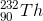What is the identity of the product following three alpha decay reactions?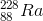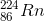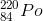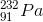Explanation:

During alpha decay, an element emits a helium nucleus with 2 neutrons and 2 protons. Thus, the atomic mass of the new element is decreased by four, and the atomic number is decreased by two.

Three subsequent alpha decays result in a new element with an atomic mass of 232 - 3(4) = 220, and a new atomic number of 90 - 3(2) = 84.

Using the periodic table, we find the element with this atomic number is polonium (Po).

### Example Question #1 : Radioactive Decay And Nuclear Chemistry

Consider the following isotope: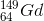What is the identity of the product after the following series of decay reactions?

alpha decay, alpha decay, electron emission, positron emission, positron emission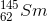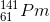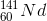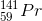Explanation:

In alpha decay, a helium nucleus is emitted, and thus the isotope loses 2 protons and 2 neutrons.

In electron emission, a neutron in the nucleus is converted into a proton and an emitted electron.

In positron emission, a proton in the nucleus is converted into a neutron and an emitted positron.

The given isotope will lose 4 protons and 4 neutrons via alpha emission, gain 1 proton and lose 1 neutron via electron emission, and lose 2 protons and gain 2 neutrons via positron emission. The result is a loss of 5 protons and 8 mass units.

Accounting for the changes in atomic mass and number, we find that the final element is 141-praseodymium.

### All GRE Subject Test: Chemistry Resources